# EMC optimized design of switching power supply PCB

The interference path of the switching converter noise provides coupling conditions for the interference source and the interfered device, and the research on common mode interference and differential mode interference is especially important. It mainly analyzes the high frequency model of the main components of the circuit and the circuit model of common mode and differential mode noise, which provides useful help for the EMC optimization design of the switching power supply PCB.

The common mode interference and differential mode interference of the switching power supply have different effects on the circuit. Generally, the low frequency time difference mode noise dominates, the common mode noise dominates at high frequency, and the common mode current radiation is usually more than the differential mode current. Radiation is much more important, so it is necessary to distinguish between differential mode interference and common mode interference in the power supply.

In order to distinguish between differential mode interference and common mode interference, we first need to study the basic coupling mode of the switching power supply. On this basis, we can establish the circuit path of differential mode noise current and common mode noise current. The conduction coupling of switching power supplies mainly includes:

Circuitry conduction coupling, capacitive coupling, inductive coupling, and a mixture of these coupling methods.

1 Common mode and differential mode noise path model

In the switching power supply, due to the coupling capacitance CW existing between the primary and secondary windings of the high-frequency transformer, the stray capacitance CK existing between the power tube and the heat sink, the parasitic parameters of the power tube itself, and the printed wiring are mutually coupled. The parasitic parameters such as mutual inductance, self-inductance, mutual capacitance, self-capacity, and impedance constitute a common mode noise and a differential mode noise path, thereby forming common mode and differential mode conduction interference. Based on the analysis of the parasitic parameters of the power switching device, the transformer and the resistance, inductance and capacitance of the printed conductor, the noise current path model of the converter can be obtained.

2 high frequency model of the main components of the circuit

The internal parasitic inductance and capacitance of the power switch tube affect the high-frequency performance of the circuit. These capacitors cause high-frequency interference leakage current to flow to the metal substrate, and there is a stray capacitance CK between the power tube and the heat sink for safety reasons. The heat sink is usually grounded, which provides a common mode noise path.

When the PWM converter is operating, along with the operation of the switching device, common mode noise is also generated. As shown in Figure 1, for the half-bridge converter, the drain voltage of the switch Q1 is always U1, and the source potential varies between 0 and U1/2 as the switch state changes; the source potential of Q2 is always 0. The drain potential varies between 0 and U1/2. In order to keep the switch tube and the heat sink in good contact, an insulating gasket is often added between the bottom of the switch tube and the heat sink or an insulating silica gel with good thermal conductivity is applied. This makes it equivalent to the presence of a parallel coupling capacitor CK between point A and ground. When the state of the switching transistors Q1 and Q2 changes and the potential at point A changes, a noise current Ick is generated on CK, as shown in Fig. 2. The current reaches the casing by the heat sink, and the casing, that is, the earth and the main power line, has a coupling impedance, forming a common mode noise path shown by a broken line in FIG. Thus, the common mode noise current produces a voltage drop across the coupling impedance Z of the ground and the main power supply line, forming common mode noise.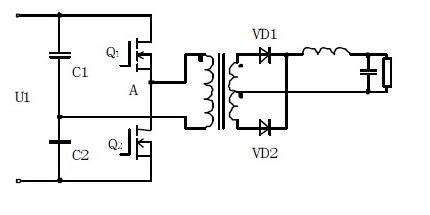Figure 1 Schematic diagram of the half-bridge converter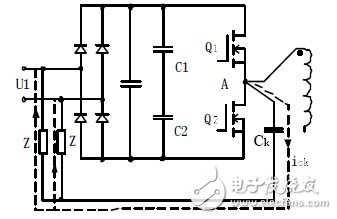Figure 2 Common mode current loop formed by the capacitance of the switch tube to ground

The isolation transformer is a widely used power line interference suppression measure. Its basic function is to achieve electrical isolation between circuits, to solve the mutual interference between devices caused by the ground loop. For an ideal transformer, it can only transmit differential mode currents and cannot transmit common mode currents because, for common mode currents, it has the same potential between the two terminals of an ideal transformer, so it cannot generate a magnetic field on the windings. It is impossible to have a common mode current path, thereby suppressing common mode noise.

The actual isolation transformer has a coupling capacitor CW between the primary and secondary sides. This coupling capacitor is due to the presence of non-dielectric and physical gaps between the windings of the transformer, which provides a path for common mode current.

As shown in Figure 2, point A is the area where the voltage change is the strongest in the circuit, and it is also the strongest area where noise is generated. Along with the high-frequency switching operation of the circuit, the high-frequency voltage at this point passes through the distributed capacitance Cps between the primary and secondary of the transformer, the impedance of the power line to the ground, the impedance of the transformer secondary line itself, the inductance, the capacitance, and the like. The common mode noise path of the transformer is formed.

The common isolation transformer has a certain suppression effect on the common mode noise, but the suppression effect on the common mode interference decreases with the increase of the frequency due to the distributed capacitance between the windings. The suppression of common mode interference by a common isolation transformer can be estimated by the ratio of the distributed capacitance between the primary and secondary and the distributed capacitance of the device to the ground. Generally, the distributed capacitance between the primary and secondary is several hundred pF, and the distributed capacitance of the device to the ground is several to several tens of nF, so the attenuation value of the common mode interference is about 10 to 20 times, that is, 20 to 30 dB. In order to improve the isolation transformer's ability to suppress common mode noise, the key is to have a small coupling capacitor. For this reason, a shield layer can be added between the primary and secondary of the transformer. The shielding layer has no adverse effect on the energy transfer of the transformer, but affects the coupling capacitance between the windings. In addition to suppressing common mode interference, the shielded transformer can also suppress differential mode interference by using a shield layer. The specific method is to connect the transformer shield to the primary center line. For the 50Hz power frequency signal, since the primary and shielding layers have a high capacitive reactance, they can still be transmitted to the secondary through the transformer effect without being attenuated. For higher frequency differential mode interference, since the capacitive reactance between the primary and the shield layer becomes smaller, this part of the interference is directly returned to the grid via the distribution capacitor and the wiring of the shield layer and the primary neutral line end, without entering the secondary loop.

Therefore, it is very important to model the high frequency of the transformer, especially the many parasitic parameters of the transformer, such as leakage inductance, distributed capacitance between the primary and secondary sides, etc., which have a significant impact on the level of common mode EMI. Must be considered. In practice, the main parameters of the transformer can be measured using an impedance measuring device to obtain these parameters and perform simulation analysis.

The DC electrolytic capacitor Cin in the half-bridge circuit has a corresponding series equivalent inductance ESL and series equivalent resistance. These two parameters also affect the high frequency performance of the circuit. In general, the ESL is about several tens of nH. In the actual analysis, the high-frequency equivalent parasitic parameters of passive components such as resistors, inductors and capacitors can be measured by a high-frequency impedance analyzer. The high-frequency model of the power device can be obtained from the model library of the circuit simulation software.

Another factor that has a high impact on the high-frequency noise of the circuit is the mutual coupling of printed conductors (strip lines) on the printed board. When a high-amplitude transient current or rapidly rising voltage appears near the signal-carrying In the vicinity of the conductor, interference problems will occur. The coupling of printed conductors is usually characterized by the mutual capacitance and mutual inductance of the circuit and the conductor. The capacitive coupling induces a coupling current, and the inductive coupling induces a coupling voltage. The parameters of the PCB layer, the traces of the signal lines, and the spacing between them affect these parameters.

The main difficulty in establishing a high-frequency model of a printed circuit board trace and extracting parasitic parameters between traces is to determine the capacitance per unit length of the printed board line and the inductance per unit length. There are usually three ways to determine the inductor and capacitor matrix components:

(1) Finite Difference Method (FDM); (2) Finite Element Method (FEM); (3) Momentum Method (MOM).

After the unit length matrix is ​​accurately determined, a high-frequency simulation model of the printed circuit board trace can be obtained by a multi-conductor transmission line or a partial element equivalent circuit (PEE C) theory. Cadence software is a powerful EDA software with SpecctraQuest tools for signal integrity and electromagnetic compatibility analysis of PCBs. It can also be used to model high-frequency printed circuit board traces to achieve a PCB for a given structure. Parameter extraction is performed, and parasitic parameter matrices such as inductance, capacitance, and resistance of any shape printed conductor trace are generated, and then EMC simulation analysis can be performed by using PEEC theory.

3 Common mode and differential mode noise circuit model

Common mode interference and differential mode interference are common in the circuit. Common mode interference exists between any phase line and ground of the power supply. Differential mode interference exists between the phase line and the phase line. Teuling, Schnaen and Roudet of the Grenoble Electrotechnical Laboratory in France based on a 400W MOSFET-based chopper circuit experimental model with a switching frequency of 100KHz showed that low-frequency time-difference mode interference dominates; at high frequencies, common-mode interference dominates. Status, which indicates that the differential mode interference and common mode interference of the switching power supply have different effects on the circuit; on the other hand, the influence of the line parasitic parameters on differential mode interference and common mode interference is also different, due to the impedance and line between the lines— ——The ground impedance is different. After the long-distance transmission, the attenuation of the differential mode component is larger than the common mode. Therefore, in order to solve the conduction noise problem of the switching power supply, it is necessary to first distinguish between common mode and differential mode interference. This requires establishing common mode and differential mode noise paths, and then simulating and analyzing them separately. This method is convenient for us to find electromagnetic interference. The root of the problem is easy to solve the problem.

In the engineering, the current probe can be used to judge whether the power supply is common mode or differential mode. The probe first surrounds each wire separately, and the induced value of the single wire is obtained. Then, the two wires are wrapped to detect the sensing condition. The induced value is increased, and the interference current in the line is common mode, and vice versa. In the theoretical analysis, for different systems, it is necessary to establish their common mode and differential mode noise current models separately. Based on our above analysis, considering the high-frequency model of the power device and the coupling relationship between the printed wires, we get A common mode and differential mode interference circuit model for a half-bridge QRC converter is shown in FIG. The Line Impedence Stabilizing Network (LISN) is a linear impedance fixed network specified by EMC. Because the inductance of the 50Hz power frequency signal LISN is low impedance and the capacitance is high impedance, the power frequency signal LISN is basically not attenuated, and the power supply can be transmitted to the half bridge converter via the LISN. For high-frequency noise, the inductance of the LISN appears as a large impedance, and the capacitance can be regarded as a short circuit, so the LISN prevents the transmission of high-frequency noise between the device under test and the grid. Therefore, the LISN acts as a common mode and differential mode interference. The current provides a fixed impedance (50 ohms) in the desired measurement band (typically 100 kHz to 30 MHz).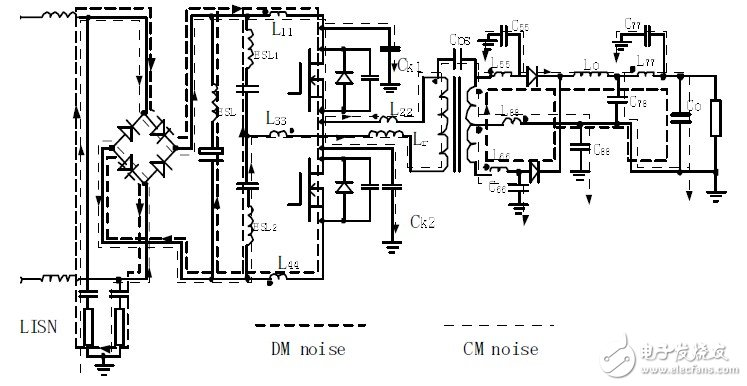Fig. 3 Noise model of half-bridge QR C converter

In the above figure, the common mode noise current starts from two sets of LISNs, and passes through the circuit switching device, the transformer, the PCB printed conductor, the secondary side circuit, and returns to the LISN to form a common mode noise current loop. The differential mode noise current forms a loop between the two sets of LISN, printed conductors, switching devices, and transformers. The common mode noise and the differential mode noise can be taken from half or the sum of the differences between the voltages of the resistors of the two sets of LISN, respectively.

which is: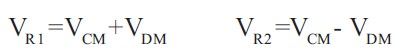and so: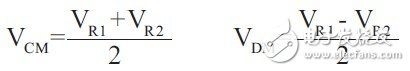In the same way, it is convenient to obtain a conductive interference circuit model of other topologies.